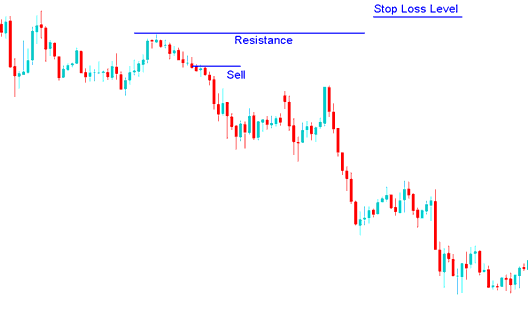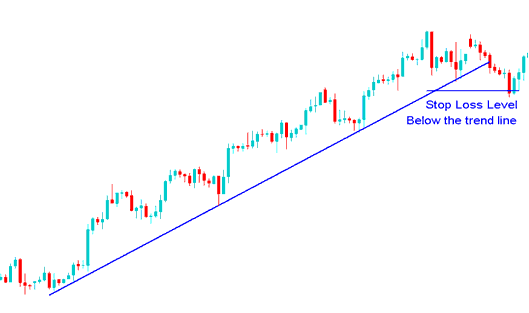# Methods of Setting Stop Loss Indices Trading Orders In Indices Trading

Traders using a indices trading system must have mathematical calculations that reveal where the order must be placed.

A Indices trader can also place a stop loss indices trading order according to the technical indicators used to set these orders. Certain technical indicators use mathematical equations to calculate where the stop loss stock indices order should be set so as to provide an optimal exit point. These indicators can be used as the basis for setting these orders.

Other indices traders also place these orders according to a predetermined risk to reward ratio. This method of setting is dependent upon certain mathematical equations. For example a ratio of 50 pips stop loss can be used by a Indices trader if the trade has the potential to make 100 pips in profit; this is a risk reward ratio of 2:1

Others just use a predetermined percentage of their total trading account balance.

To set a stop loss order it is best to use one of the following methods:

## 1. Percentage of indices trading account balance

This is based on the percent of account balance that the indices trader is willing to risk.

If a indices trader is willing to risk 2% of account balance then the indices trader determines how far he will set the order level based on the position size that he has bought or sold.

Example:

If a indices trader has a \$100,000 account and is willing to risk 2% then the position size of the trade that they will open for Indices will be determined by this 2% stop loss level.

## 2. Setting Stop Loss Indices Trading Order using Support and Resistance Levels

Another way of setting stop loss stock indices orders is to use supports and resistance levels, on the trading charts.

Given that stop loss indices trading orders tend to congregate at key points, when one of these levels is touched by the indices price, others are set off, like dominos. Stop loss orders tend to accumulate just above or below the resistance or support levels, respectively.

A resistance or a support level should act like a barrier for stock indexes price movement, this is why they are used to set stop losses, if this barrier is broken the stock indexes price movement can go towards the opposite direction of the original indices trade, but if this barriers (support and resistance levels) are not broken the stock indexes price will continue moving in the intended direction.### Stop Loss Indices Trading Order level using a resistance levelSetting order above the resistance

### Stop Loss Indices Trading Order level using a support LevelSetting order below the Support Line

## 3. Indices Trend Lines

A indices trend line can be used to set stop losses where the order is set just below the indices trend line. As long as the indices trend line holds the indices trader will be able to continue making profits while at the same time set this order that will lock his profit once the indices trend line is broken.Setting order below the indices trend line

Example of where to set this order using indices trend lines.